Documentation

# plot

Plot graph nodes and edges

## Syntax

``plot(G)``
``plot(G,LineSpec)``
``plot(___,Name,Value)``
``plot(ax,___)``
``h = plot(___)``

## Description

example

````plot(G)` plots the nodes and edges in graph `G`.```

example

````plot(G,LineSpec)` sets the line style, marker symbol, and color. For example, `plot(G,'-or')` uses red circles for the nodes and red lines for the edges.```

example

````plot(___,Name,Value)` uses additional options specified by one or more Name-Value pair arguments using any of the input argument combinations in previous syntaxes. For example, `plot(G,'Layout','circle')` plots a circular ring layout of the graph, and `plot(G,'XData',X,'YData',Y,'ZData',Z)` specifies the `(X,Y,Z)` coordinates of the graph nodes.```
````plot(ax,___)` plots into the axes specified by `ax` instead of into the current axes (`gca`). The option, `ax`, can precede any of the input argument combinations in previous syntaxes.```

example

````h = plot(___)` returns a `GraphPlot` object. Use this object to inspect and adjust the properties of the plotted graph.```

## Examples

collapse all

Create a graph using a sparse adjacency matrix, and then plot the graph.

```n = 10; A = delsq(numgrid('L',n+2)); G = graph(A,'omitselfloops')```
```G = graph with properties: Edges: [130x2 table] Nodes: [75x0 table] ```
`plot(G)`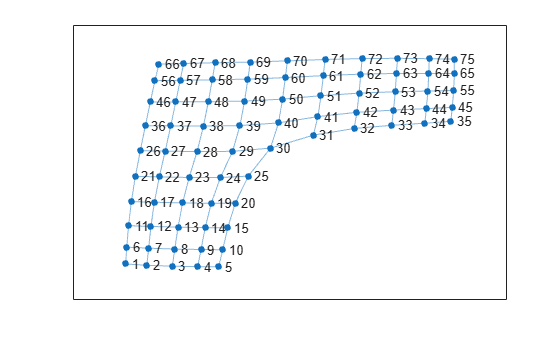Create and plot a graph. Specify the `LineSpec` input to change the `Marker`, `NodeColor`, and/or `LineStyle` of the graph plot.

```G = graph(bucky); plot(G,'-.dr','NodeLabel',{})```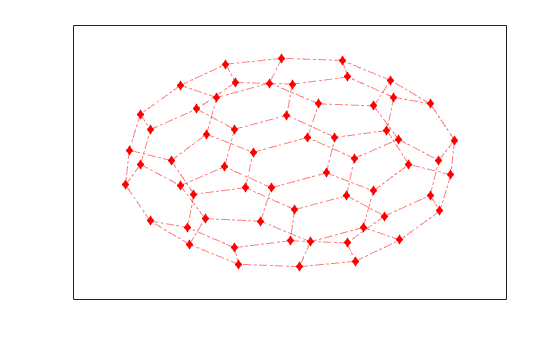Create a directed graph, and then plot the graph using the `'force'` layout.

```G = digraph(1,2:5); G = addedge(G,2,6:15); G = addedge(G,15,16:20)```
```G = digraph with properties: Edges: [19x1 table] Nodes: [20x0 table] ```
`plot(G,'Layout','force')`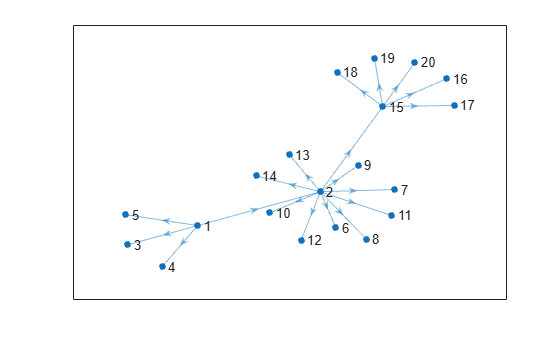Create a weighted graph.

```s = [1 1 1 1 1 2 2 7 7 9 3 3 1 4 10 8 4 5 6 8]; t = [2 3 4 5 7 6 7 5 9 6 6 10 10 10 11 11 8 8 11 9]; weights = [1 1 1 1 3 3 2 4 1 6 2 8 8 9 3 2 10 12 15 16]; G = graph(s,t,weights)```
```G = graph with properties: Edges: [20x2 table] Nodes: [11x0 table] ```

Plot the graph using custom coordinates for the nodes. The x-coordinates are specified using `XData`, the y-coordinates are specified using `YData`, and the z-coordinates are specified using `ZData`. Use `EdgeLabel` to label the edges using the edge weights.

```x = [0 0.5 -0.5 -0.5 0.5 0 1.5 0 2 -1.5 -2]; y = [0 0.5 0.5 -0.5 -0.5 2 0 -2 0 0 0]; z = [5 3 3 3 3 0 1 0 0 1 0]; plot(G,'XData',x,'YData',y,'ZData',z,'EdgeLabel',G.Edges.Weight)```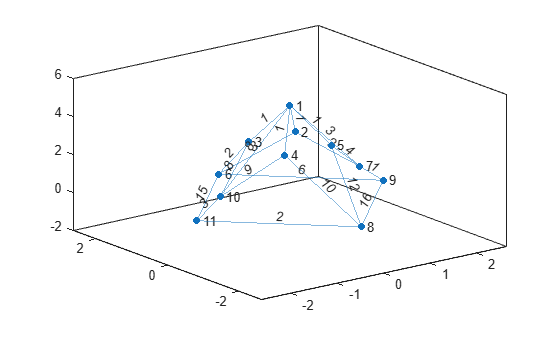View the graph from above.

`view(2)`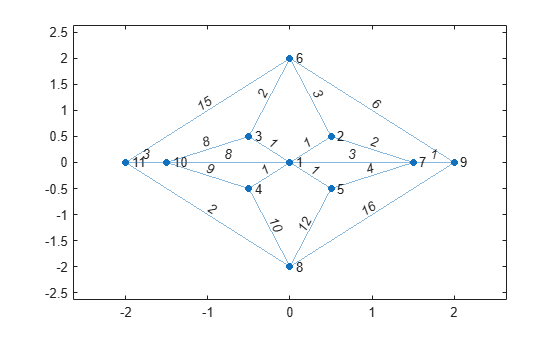Create a weighted graph.

```s = [1 1 1 1 2 2 3 4 4 5 6]; t = [2 3 4 5 3 6 6 5 7 7 7]; weights = [50 10 20 80 90 90 30 20 100 40 60]; G = graph(s,t,weights)```
```G = graph with properties: Edges: [11x2 table] Nodes: [7x0 table] ```

Plot the graph, labeling the edges with their weights, and making the width of the edges proportional to their weights. Use a rescaled version of the edge weights to determine the width of each edge, such that the widest line has a width of 5.

```LWidths = 5*G.Edges.Weight/max(G.Edges.Weight); plot(G,'EdgeLabel',G.Edges.Weight,'LineWidth',LWidths)```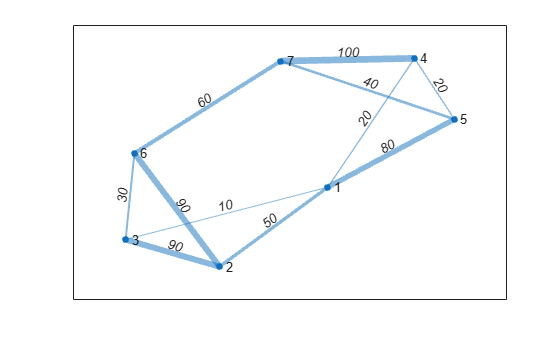Create a directed graph. Plot the graph with custom labels for the nodes and edges.

```s = [1 1 1 2 2 3 3 4 4 5 6 7]; t = [2 3 4 5 6 5 7 6 7 8 8 8]; G = digraph(s,t)```
```G = digraph with properties: Edges: [12x1 table] Nodes: [8x0 table] ```
```eLabels = {'x' 'y' 'z' 'y' 'z' 'x' 'z' 'x' 'y' 'z' 'y' 'x'}; nLabels = {'{0}','{x}','{y}','{z}','{x,y}','{x,z}','{y,z}','{x,y,z}'}; plot(G,'Layout','force','EdgeLabel',eLabels,'NodeLabel',nLabels)```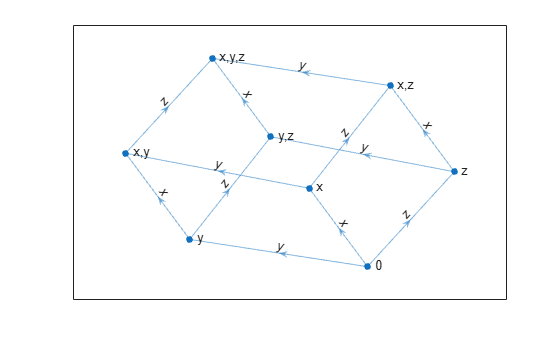Create and plot a directed graph. Specify an output argument to `plot` to return a handle to the `GraphPlot` object.

```s = [1 1 1 2 2 3 3 4 5 5 6 7 7 8 8 9 10 11]; t = [2 3 10 4 12 4 5 6 6 7 9 8 10 9 11 12 11 12]; G = digraph(s,t)```
```G = digraph with properties: Edges: [18x1 table] Nodes: [12x0 table] ```
`p = plot(G)`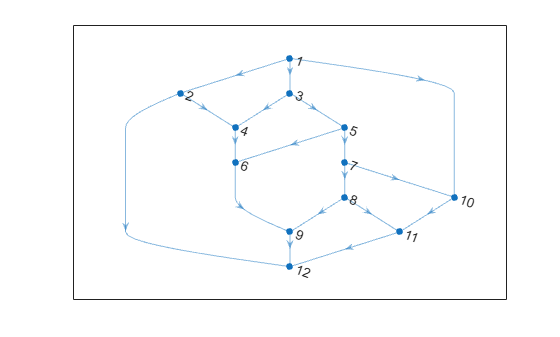```p = GraphPlot with properties: NodeColor: [0 0.4470 0.7410] MarkerSize: 4 Marker: 'o' EdgeColor: [0 0.4470 0.7410] LineWidth: 0.5000 LineStyle: '-' NodeLabel: {1x12 cell} EdgeLabel: {} XData: [2.5000 1.5000 2.5000 2 3 2 3 3 2.5000 4 3.5000 2.5000] YData: [7 6 6 5 5 4 4 3 2 3 2 1] ZData: [0 0 0 0 0 0 0 0 0 0 0 0] Show all properties ```

Change the color and marker of the nodes.

```p.Marker = 's'; p.NodeColor = 'r';```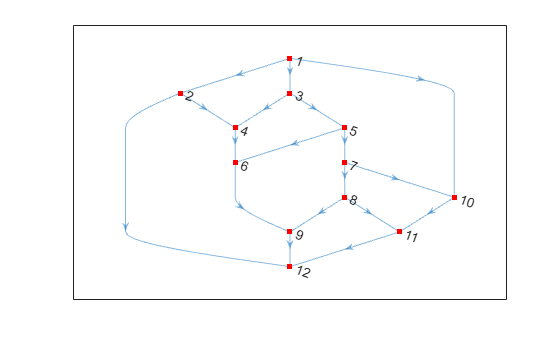Increase the size of the nodes.

`p.MarkerSize = 7;`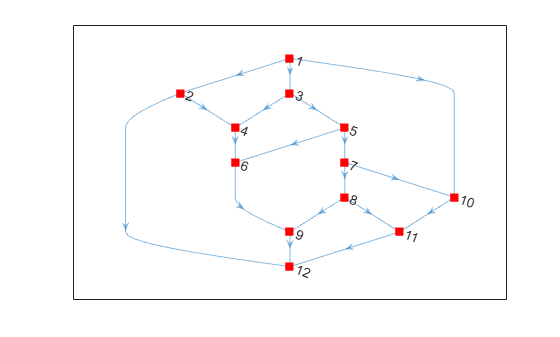Change the line style of the edges.

`p.LineStyle = '--';`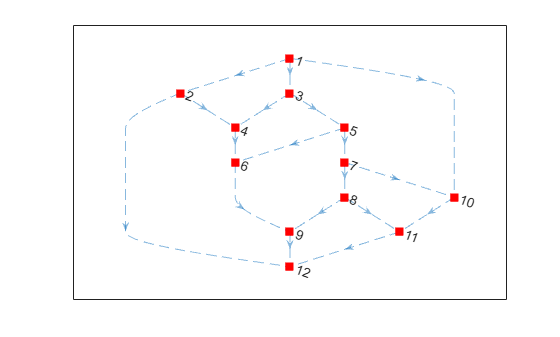Change the x and y coordinates of the nodes.

```p.XData = [2 4 1.5 3.5 1 3 1 2.1 3 2 3.1 4]; p.YData = [3 3 3.5 3.5 4 4 2 2 2 1 1 1];```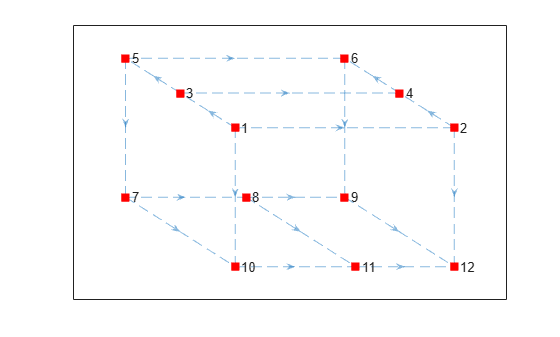## Input Arguments

collapse all

Input graph, specified as either a `graph` or `digraph` object. Use `graph` to create an undirected graph or `digraph` to create a directed graph.

Example: `G = graph(1,2)`

Example: `G = digraph([1 2],[2 3])`

Line style, marker symbol, and color, specified as a character vector or string vector of symbols. The symbols can appear in any order, and you can omit one or more of the characteristics. If you omit the line style, then the plot shows solid lines for the graph edges.

Example: `'--or'` uses red circle node markers and red dashed lines as edges.

Example: `'r*'` uses red asterisk node markers and solid red lines as edges.

SymbolLine Style
`-`Solid line (default)
`--`Dashed line
`:`Dotted line
`-.`Dash-dot line
SymbolMarker
`o`Circle
`+`Plus sign
`*`Asterisk
`.`Point
`x`Cross
`s`Square
`d`Diamond
`^`Upward-pointing triangle
`v`Downward-pointing triangle
`>`Right-pointing triangle
`<`Left-pointing triangle
`p`Pentagram
`h`Hexagram
SymbolColor

`y`

yellow

`m`

magenta

`c`

cyan

`r`

red

`g`

green

`b`

blue

`w`

white

`k`

black

Axes object. If you do not specify an axes object, then `plot` uses the current axes (`gca`).

### Name-Value Pair Arguments

Specify optional comma-separated pairs of `Name,Value` arguments. `Name` is the argument name and `Value` is the corresponding value. `Name` must appear inside quotes. You can specify several name and value pair arguments in any order as `Name1,Value1,...,NameN,ValueN`.

Example: ```p = plot(G,'EdgeColor','r','NodeColor','k','LineStyle','--')```

The graph properties listed here are only a subset. For a complete list, see GraphPlot Properties.

### Note

`ArrowSize` only affects the display of directed graphs created using `digraph`.

Arrow size, specified as the comma-separated pair consisting of `'ArrowSize'` and a positive value in point units. The default value of `ArrowSize` is `7` for graphs with 100 or fewer nodes, and `4` for graphs with more than 100 nodes.

Example: `15`

Color data of edge lines, specified as the comma-separated pair consisting of `'EdgeCData'` and a vector with length equal to the number of edges in the graph. The values in `EdgeCData` map linearly to the colors in the current colormap, resulting in different colors for each edge in the plotted graph.

Edge color, specified as the comma-separated pair consisting of `'EdgeColor'` and one of these values:

• `'none'` — Edges are not drawn.

• `'flat'` — Color of each edge depends on the value of `EdgeCData`.

• matrix — Each row is an RGB triplet representing the color of one edge. The size of the matrix is `numedges(G)`-by-`3`.

• RGB triplet, hexadecimal color code, or color name — Edges use the specified color.

RGB triplets and hexadecimal color codes are useful for specifying custom colors.

• An RGB triplet is a three-element row vector whose elements specify the intensities of the red, green, and blue components of the color. The intensities must be in the range `[0,1]`; for example,``` [0.4 0.6 0.7]```.

• A hexadecimal color code is a character vector or a string scalar that starts with a hash symbol (`#`) followed by three or six hexadecimal digits, which can range from `0` to `F`. The values are not case sensitive. Thus, the color codes `'#FF8800'`, `'#ff8800'`, `'#F80'`, and `'#f80'` are equivalent.

Alternatively, you can specify some common colors by name. This table lists the named color options, the equivalent RGB triplets, and hexadecimal color codes.

Color NameShort NameRGB TripletHexadecimal Color CodeAppearance
`'red'``'r'``[1 0 0]``'#FF0000'``'green'``'g'``[0 1 0]``'#00FF00'``'blue'``'b'``[0 0 1]``'#0000FF'``'cyan'` `'c'``[0 1 1]``'#00FFFF'``'magenta'``'m'``[1 0 1]``'#FF00FF'``'yellow'``'y'``[1 1 0]``'#FFFF00'``'black'``'k'``[0 0 0]``'#000000'``'white'``'w'``[1 1 1]``'#FFFFFF'`Here are the RGB triplets and hexadecimal color codes for the default colors MATLAB® uses in many types of plots.

RGB TripletHexadecimal Color CodeAppearance
`[0 0.4470 0.7410]``'#0072BD'``[0.8500 0.3250 0.0980]``'#D95319'``[0.9290 0.6940 0.1250]``'#EDB120'``[0.4940 0.1840 0.5560]``'#7E2F8E'``[0.4660 0.6740 0.1880]``'#77AC30'``[0.3010 0.7450 0.9330]``'#4DBEEE'``[0.6350 0.0780 0.1840]``'#A2142F'`Example: `plot(G,'EdgeColor','r')` creates a graph plot with red edges.

Edge labels, specified as the comma-separated pair consisting of `'EdgeLabel'` and a numeric vector, cell array of character vectors, or string array. The length of `EdgeLabel` must be equal to the number of edges in the graph. By default `EdgeLabel` is an empty cell array (no edge labels are displayed).

Example: `{'A', 'B', 'C'}`

Example: `[1 2 3]`

Example: `plot(G,'EdgeLabel',G.Edges.Weight)` labels the graph edges with their weights.

Data Types: `single` | `double` | `int8` | `int16` | `int32` | `int64` | `uint8` | `uint16` | `uint32` | `uint64` | `cell` | `string`

Graph layout method, specified as the comma-separated pair consisting of `'Layout'` and one of the options in the table. The table also lists compatible name-value pairs to further refine each layout method. See the `layout` reference page for more information on these layout-specific name-value pairs.

OptionDescriptionLayout-Specific Name-Value Pairs
`'auto'` (default)

Automatic choice of layout method based on the size and structure of the graph.

`'circle'`

Circular layout. Places the graph nodes on a circle centered at the origin with radius 1.

`'Center'` — Center node in circular layout

`'force'`

Force-directed layout . Uses attractive forces between adjacent nodes and repulsive forces between distant nodes.

`'Iterations'` — Number of force-directed layout iterations

`'WeightEffect'` — Effect of edge weights on layout

`'UseGravity'` — Gravity toggle for layouts with multiple components

`'XStart'` — Starting x-coordinates for nodes

`'YStart'` — Starting y-coordinates for nodes

`'layered'`

Layered node layout , , . Places the graph nodes into a set of layers, revealing hierarchical structure. By default the layers progress downwards (the arrows of a directed acyclic graph point down).

`'Direction'` — Direction of layers

`'Sources'` — Nodes to include in the first layer

`'Sinks'` — Nodes to include in the last layer

`'AssignLayers'` — Layer assignment method

`'subspace'`

Subspace embedding node layout . Plots the graph nodes in a high-dimensional embedded subspace, and then projects the positions back into 2-D. By default the subspace dimension is either 100 or the total number of nodes, whichever is smaller.

`'Dimension'` — Dimension of embedded subspace

`'force3'`3-D force-directed layout.

`'Iterations'` — Number of force-directed layout iterations

`'WeightEffect'` — Effect of edge weights on layout

`'UseGravity'` — Gravity toggle for layouts with multiple components

`'XStart'` — Starting x-coordinates for nodes

`'YStart'` — Starting y-coordinates for nodes

`'ZStart'` — Starting z-coordinates for nodes

`'subspace3'`3-D subspace embedding layout.

`'Dimension'` — Dimension of embedded subspace

Example: `plot(G,'Layout','force3','Iterations',10)`

Example: `plot(G,'Layout','subspace','Dimension',50)`

Example: `plot(G,'Layout','layered')`

Line style, specified as the comma-separated pair consisting of `'LineStyle'` and one of the line styles listed in this table, or as a cell array or string vector of such values. Specify a cell array of character vectors or string vector to use different line styles for each edge.

Character(s)Line StyleResulting Line
`'-'`Solid line`'--'`Dashed line`':'`Dotted line`'-.'`Dash-dotted line`'none'`No lineNo line

Edge line width, specified as the comma-separated pair consisting of `'LineWidth'` and a positive value in point units or a vector of such values. Specify a vector to use a different line width for each edge in the graph.

Example: `0.75`

Node marker symbol, specified as the comma-separated pair consisting of `'Marker'` and one of the character vectors listed in this table, or as a cell array or string vector of such values. The default is to use circular markers for the graph nodes. Specify a cell array of character vectors or string vector to use different markers for each node.

ValueDescription
`'o'`Circle
`'+'`Plus sign
`'*'`Asterisk
`'.'`Point
`'x'`Cross
`'square'` or `'s'`Square
`'diamond'` or `'d'`Diamond
`'^'`Upward-pointing triangle
`'v'`Downward-pointing triangle
`'>'`Right-pointing triangle
`'<'`Left-pointing triangle
`'pentagram'` or `'p'`Five-pointed star (pentagram)
`'hexagram'` or `'h'`Six-pointed star (hexagram)
`'none'`No markers

Example: `'+'`

Example: `'diamond'`

Node marker size, specified as the comma-separated pair consisting of `'MarkerSize'` and a positive value in point units or as a vector of such values. Specify a vector to use different marker sizes for each node in the graph. The default value of `MarkerSize` is 4 for graphs with 100 or fewer nodes, and `2` for graphs with more than 100 nodes.

Example: `10`

Color data of node markers, specified as the comma-separated pair consisting of `'NodeCData'` and a vector with length equal to the number of nodes in the graph. The values in `NodeCData` map linearly to the colors in the current colormap, resulting in different colors for each node in the plotted graph.

Node color, specified as the comma-separated pair consisting of `'NodeColor'` and one of these values:

• `'none'` — Nodes are not drawn.

• `'flat'` — Color of each node depends on the value of `NodeCData`.

• matrix — Each row is an RGB triplet representing the color of one node. The size of the matrix is `numnodes(G)`-by-`3`.

• RGB triplet, hexadecimal color code, or color name — Nodes use the specified color.

RGB triplets and hexadecimal color codes are useful for specifying custom colors.

• An RGB triplet is a three-element row vector whose elements specify the intensities of the red, green, and blue components of the color. The intensities must be in the range `[0,1]`; for example,``` [0.4 0.6 0.7]```.

• A hexadecimal color code is a character vector or a string scalar that starts with a hash symbol (`#`) followed by three or six hexadecimal digits, which can range from `0` to `F`. The values are not case sensitive. Thus, the color codes `'#FF8800'`, `'#ff8800'`, `'#F80'`, and `'#f80'` are equivalent.

Alternatively, you can specify some common colors by name. This table lists the named color options, the equivalent RGB triplets, and hexadecimal color codes.

Color NameShort NameRGB TripletHexadecimal Color CodeAppearance
`'red'``'r'``[1 0 0]``'#FF0000'``'green'``'g'``[0 1 0]``'#00FF00'``'blue'``'b'``[0 0 1]``'#0000FF'``'cyan'` `'c'``[0 1 1]``'#00FFFF'``'magenta'``'m'``[1 0 1]``'#FF00FF'``'yellow'``'y'``[1 1 0]``'#FFFF00'``'black'``'k'``[0 0 0]``'#000000'``'white'``'w'``[1 1 1]``'#FFFFFF'`Here are the RGB triplets and hexadecimal color codes for the default colors MATLAB uses in many types of plots.

RGB TripletHexadecimal Color CodeAppearance
`[0 0.4470 0.7410]``'#0072BD'``[0.8500 0.3250 0.0980]``'#D95319'``[0.9290 0.6940 0.1250]``'#EDB120'``[0.4940 0.1840 0.5560]``'#7E2F8E'``[0.4660 0.6740 0.1880]``'#77AC30'``[0.3010 0.7450 0.9330]``'#4DBEEE'``[0.6350 0.0780 0.1840]``'#A2142F'`Example: `plot(G,'NodeColor','k')` creates a graph plot with black nodes.

Node labels, specified as the comma-separated pair consisting of `'NodeLabel'` and a numeric vector, cell array of character vectors, or string array. The length of `NodeLabel` must be equal to the number of nodes in the graph. By default `NodeLabel` is a cell array containing the node IDs for the graph nodes:

• For nodes without names (that is, `G.Nodes` does not contain a `Name` variable), the node labels are the values `unique(G.Edges.EndNodes)` contained in a cell array.

• For named nodes, the node labels are `G.Nodes.Name'`.

Example: `{'A', 'B', 'C'}`

Example: `[1 2 3]`

Example: `plot(G,'NodeLabel',G.Nodes.Name)` labels the nodes with their names.

Data Types: `single` | `double` | `int8` | `int16` | `int32` | `int64` | `uint8` | `uint16` | `uint32` | `uint64` | `cell` | `string`

### Note

`XData` and `YData` must be specified together so that each node has a valid (x,y) coordinate. Optionally, you can also specify `ZData` for 3-D coordinates.

x-coordinate of nodes, specified as the comma-separated pair consisting of `'XData'` and a vector with length equal to the number of nodes in the graph.

### Note

`XData` and `YData` must be specified together so that each node has a valid (x,y) coordinate. Optionally, you can also specify `ZData` for 3-D coordinates.

y-coordinate of nodes, specified as the comma-separated pair consisting of `'YData'` and a vector with length equal to the number of nodes in the graph.

### Note

`XData` and `YData` must be specified together so that each node has a valid (x,y) coordinate. Optionally, you can also specify `ZData` for 3-D coordinates.

z-coordinate of nodes, specified as the comma-separated pair consisting of `'ZData'` and a vector with length equal to the number of nodes in the graph.

## Output Arguments

collapse all

Graph plot, returned as an object. For more information, see `GraphPlot`.

## Compatibility Considerations

expand all

Behavior changed in R2018a

 Fruchterman, T., and E. Reingold. “Graph Drawing by Force-directed Placement.” Software — Practice & Experience. Vol. 21 (11), 1991, pp. 1129–1164.

 Gansner, E., E. Koutsofios, S. North, and K.-P Vo. “A Technique for Drawing Directed Graphs.” IEEE Transactions on Software Engineering. Vol.19, 1993, pp. 214–230.

 Barth, W., M. Juenger, and P. Mutzel. “Simple and Efficient Bilayer Cross Counting.” Journal of Graph Algorithms and Applications. Vol.8 (2), 2004, pp. 179–194.

 Brandes, U., and B. Koepf. “Fast and Simple Horizontal Coordinate Assignment.” LNCS. Vol. 2265, 2002, pp. 31–44.

 Y. Koren. “Drawing Graphs by Eigenvectors: Theory and Practice.” Computers and Mathematics with Applications. Vol. 49, 2005, pp. 1867–1888.

Download ebook